• 计算负反馈放大电路的电压放大倍数集成运放的负反馈电路分立元件的负反馈电路 集成运放的负反馈电路 集成运放是理想运放，满足“虚短”、“虚断”的使用条件。 在计算电压放大倍数时，有两种方法：一是建立回路方程...

集成运放的负反馈电路

集成运放是理想运放，满足“虚短”、“虚断”的使用条件。

在计算电压放大倍数时，有两种方法：一是建立回路方程等式，二是计算反馈系数进而计算电压放大倍数

下面通过鲜活的实例展开说明
实例一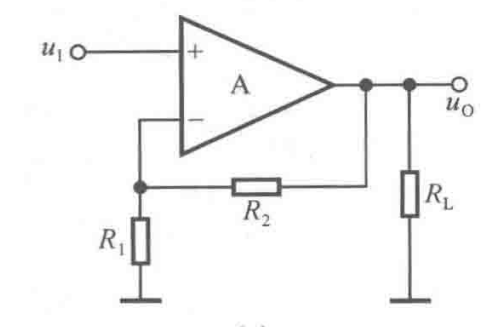法一：
由“虚短”知， U N = U P = U I

展开全文经验分享
• 文章目录A 负反馈放大电路的方框图及放大倍数的估算A.a 负反馈放大电路的方框图A.b 负反馈放大电路放大倍数的一般表达式A.c 深度负反馈的实质A.d 基于反馈系数的放大倍数的估算方法A.e 基于理想运放的放大倍数的计算...

A 负反馈放大电路的方框图及放大倍数的估算

A.a 负反馈放大电路的方框图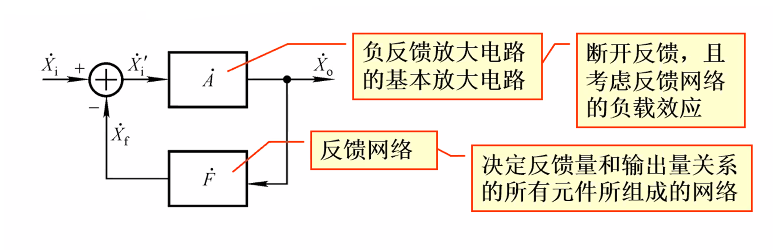方框图中信号是单向流通的。

基本（开环）放大电路的放大倍数（输出量与净输入量之比）： A ˙ = X ˙ o X i ‘ ˙ \dot{A}=\frac{\dot{X}_o}{\dot{X_i‘}}
反馈系数(反馈量与输出量之比)： F = X ˙ f X ˙ o F=\frac{\dot{X}_f}{\dot{X}_o}
反馈(闭环)放大电路的放大倍数（输出量比输入量）： A ˙ f = X ˙ o X ˙ i \dot{A}_f=\frac{\dot{X}_o}{\dot{X}_i}

A.b 负反馈放大电路放大倍数的一般表达式

反馈组态功能 A ˙ \dot{A} F ˙ \dot{F} A ˙ f \dot{A}_f
电压串联电压控制电压 U ˙ o / U ˙ i ′ \dot{U}_o/\dot{U}_i' U ˙ f / U ˙ o \dot{U}_f/\dot{U}_o U ˙ o / U ˙ i \dot{U}_o/\dot{U}_i (电压放大倍数)
电压并联电流控制电压 U ˙ o / I ˙ i ′ \dot{U}_o/\dot{I}_i' I ˙ f / U ˙ o \dot{I}_f/\dot{U}_o U ˙ o / I ˙ i （ 互 阻 放 大 倍 数 ） \dot{U}_o/\dot{I}_i（互阻放大倍数）
电流串联电压控制电流 I ˙ o / U ˙ i ′ \dot{I}_o/\dot{U}_i' U ˙ f / I ˙ o \dot{U}_f/\dot{I}_o I ˙ o / U ˙ i \dot{I}_o/\dot{U}_i （互导放大倍数）
电流并联电流控制电流 I ˙ o / I ˙ i ′ \dot{I}_o/\dot{I}_i' I ˙ f / I ˙ o \dot{I}_f/\dot{I}_o I ˙ o / I ˙ i \dot{I}_o/\dot{I}_i （电流放大倍数）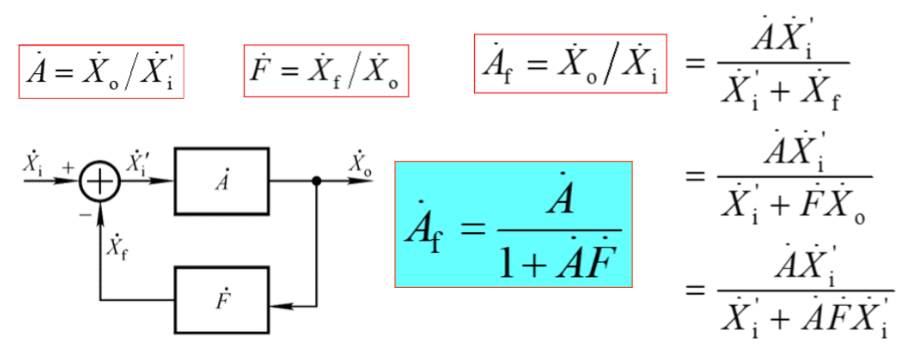A ˙ f \dot{A}_f 称为负反馈放大倍数的一般表达式。

A.c 深度负反馈的实质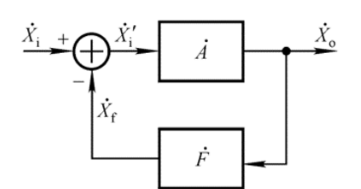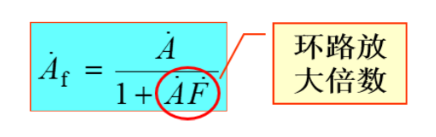只有环路放大倍数>0时，电路引入的才为负反馈（输出降低，放大倍数降低）。

深度负反馈条件：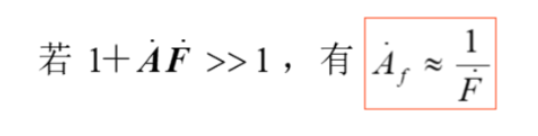表明在深度负反馈条件下，放大倍数仅决定于负反馈网络，而与基本放大电路无关，因此放大倍数具有很大高的稳定性（基本放大电路中的半导体受温度影响。）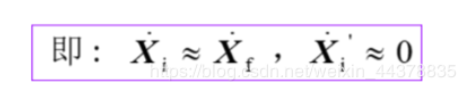上式说明：输入信号等于反馈信号，净输入信号忽略不计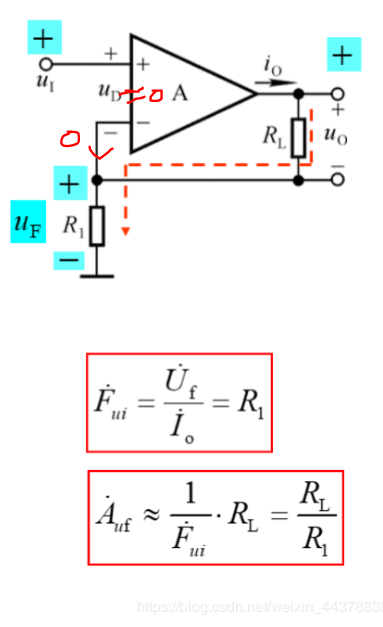• 在串联负反馈电路中， U ˙ i ≈ U ˙ f ， U ˙ i ′ ≈ 0 \dot{U}_i\approx \dot{U}_f，\dot{U}_i'\approx0
• 在并联负反馈电路中， I ˙ i ≈ I ˙ f ， I ˙ i ′ ≈ 0 \dot{I}_i\approx\dot{I}_f，\dot{I}_i'\approx 0

在中频段，通常， A ˙ 、 F ˙ 、 A ˙ f \dot{A}、\dot{F}、\dot{A}_f 符号相同。

A.d 基于反馈系数的放大倍数的估算方法

交流负反馈的阻态 → F ˙ → ( 利 用 深 度 负 反 馈 ) A ˙ u f \rightarrow\dot{F}\rightarrow(利用深度负反馈) \dot{A}_{uf}

A.d.a 电压串联负反馈电路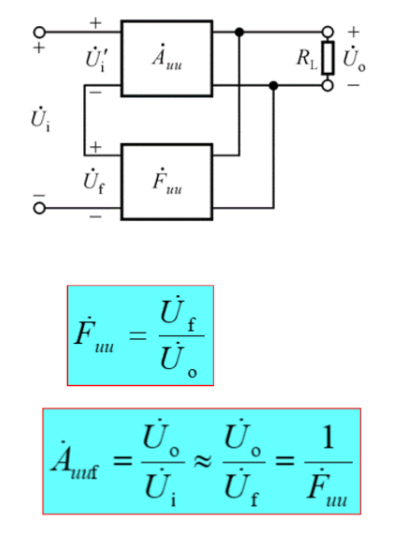例子：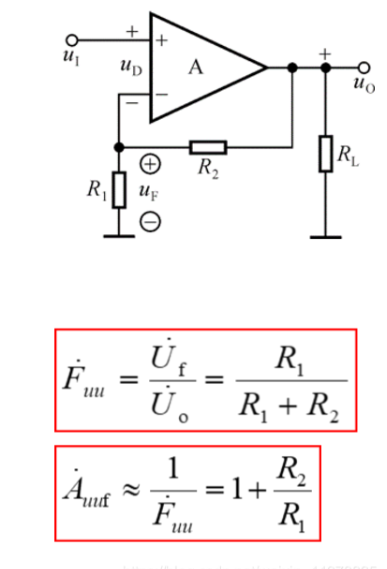A.d.b 电压并联负反馈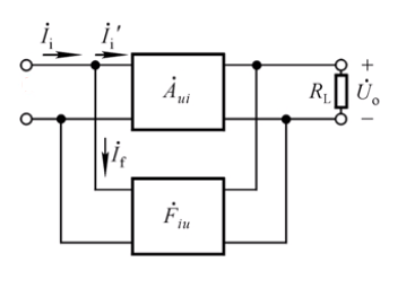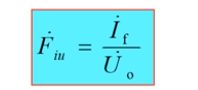加含有内阻的电压源。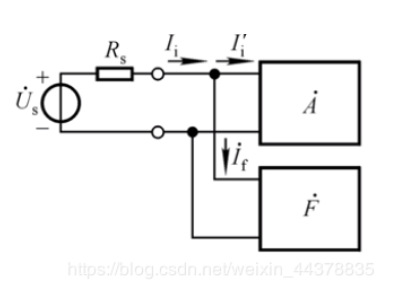深度负反馈时 I ’ i ≈ . 0 I’_i\approx. 0 ，则 U ˙ I ≈ 0 \dot{U}_I\approx 0 所以用 U ˙ s \dot{U}_s 代替 U ˙ i 且 U ˙ s \dot{U}_i且\dot{U}_s 两端电压几乎在 R s R_s 两端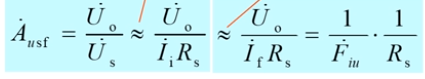例子：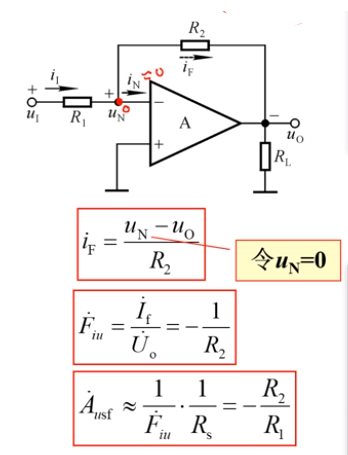A.d.c 电流串联负反馈电流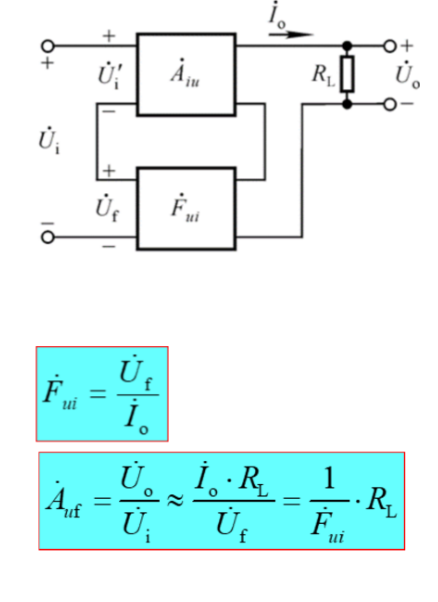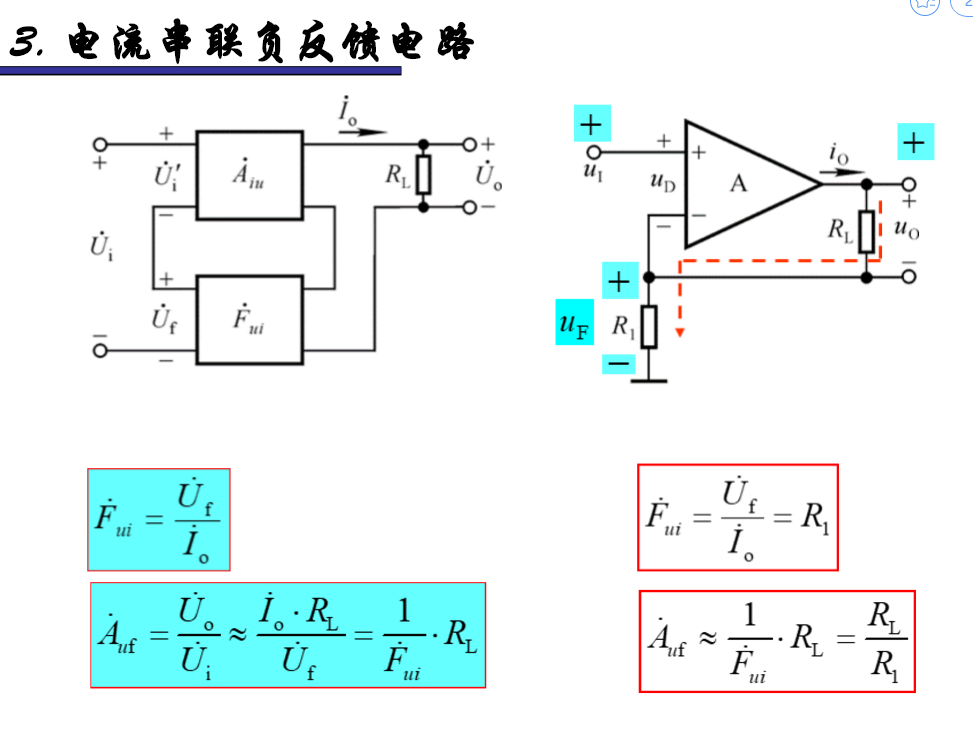例子：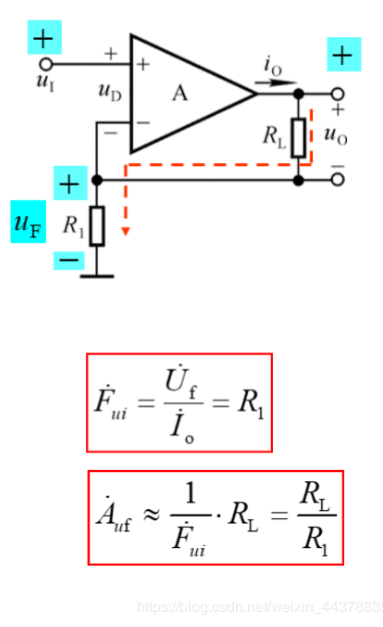A.d.d 电流并联负反馈电路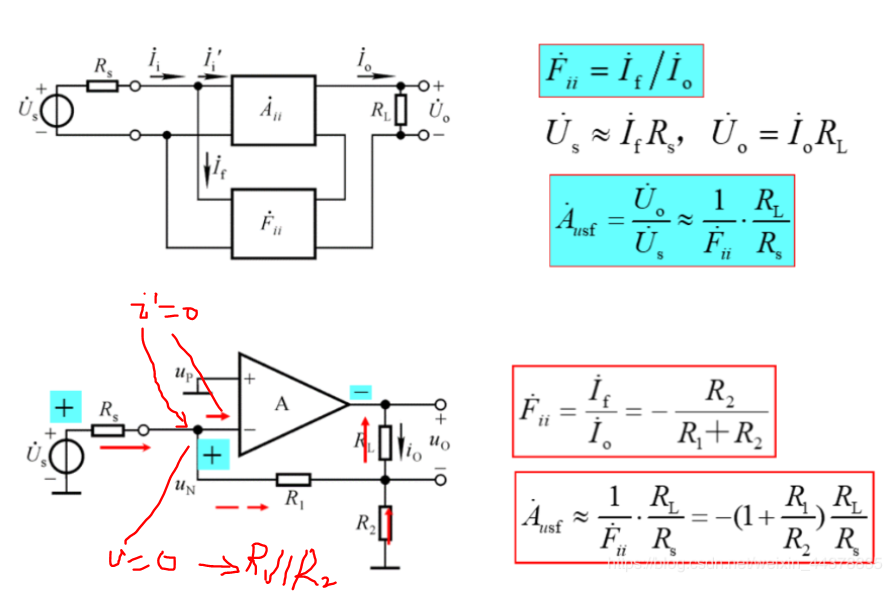小结：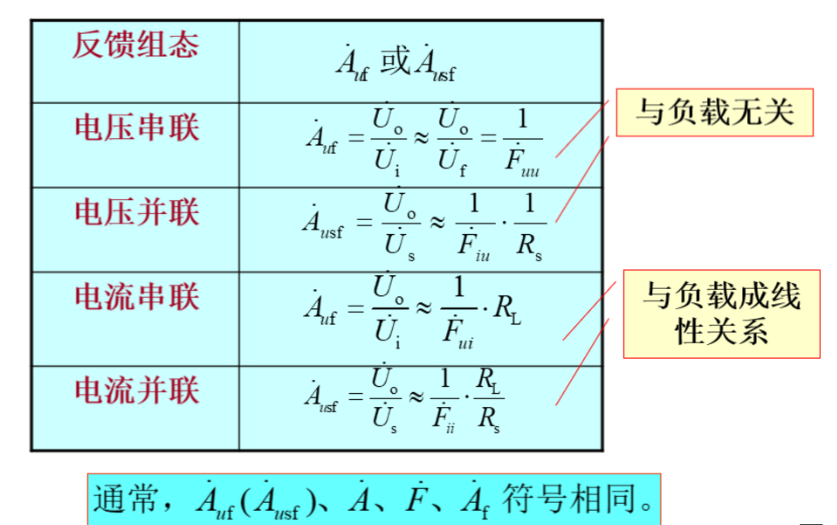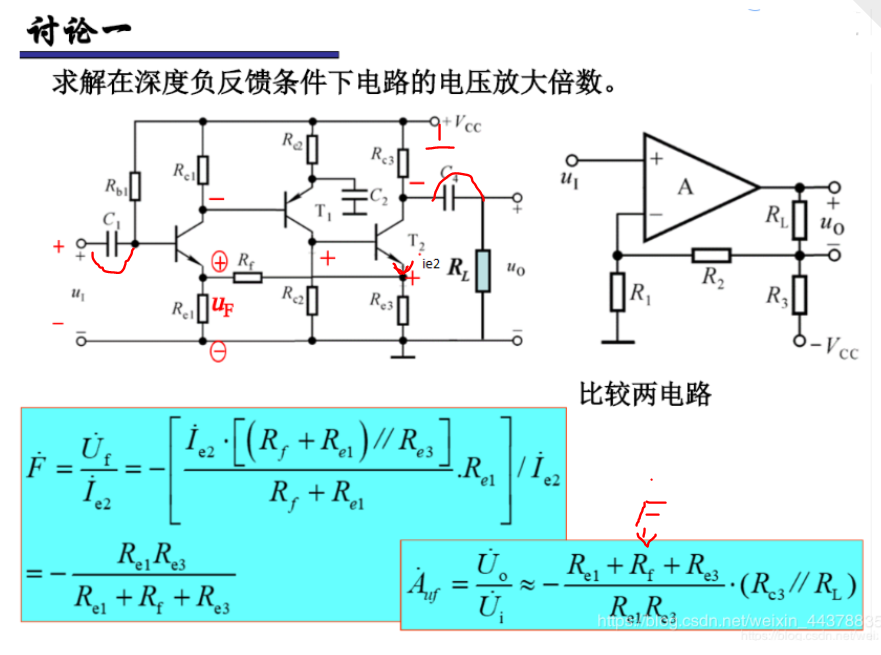A.e 基于理想运放的放大倍数的计算方法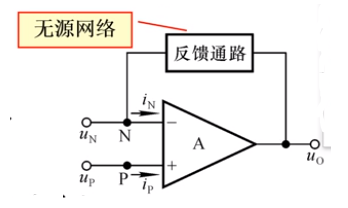1 理想运放参数特点（大的参数趋近于无穷大，小的参数趋近于0）：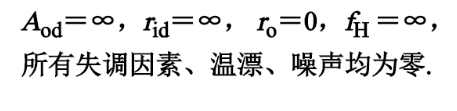2 理想运放工作在线性区的电路特征：

引入负反馈。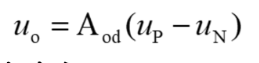3 理想运放工作在线性区的特点：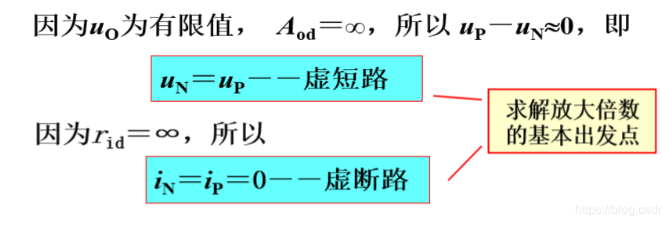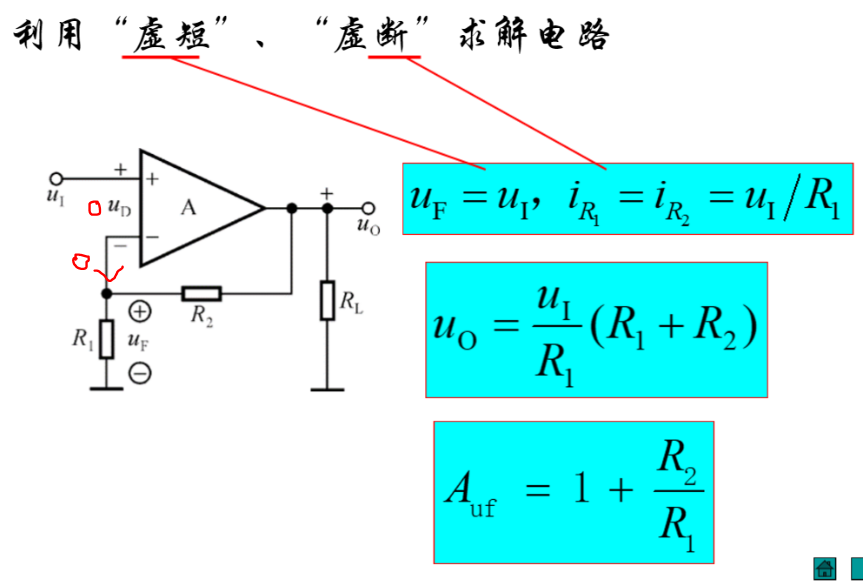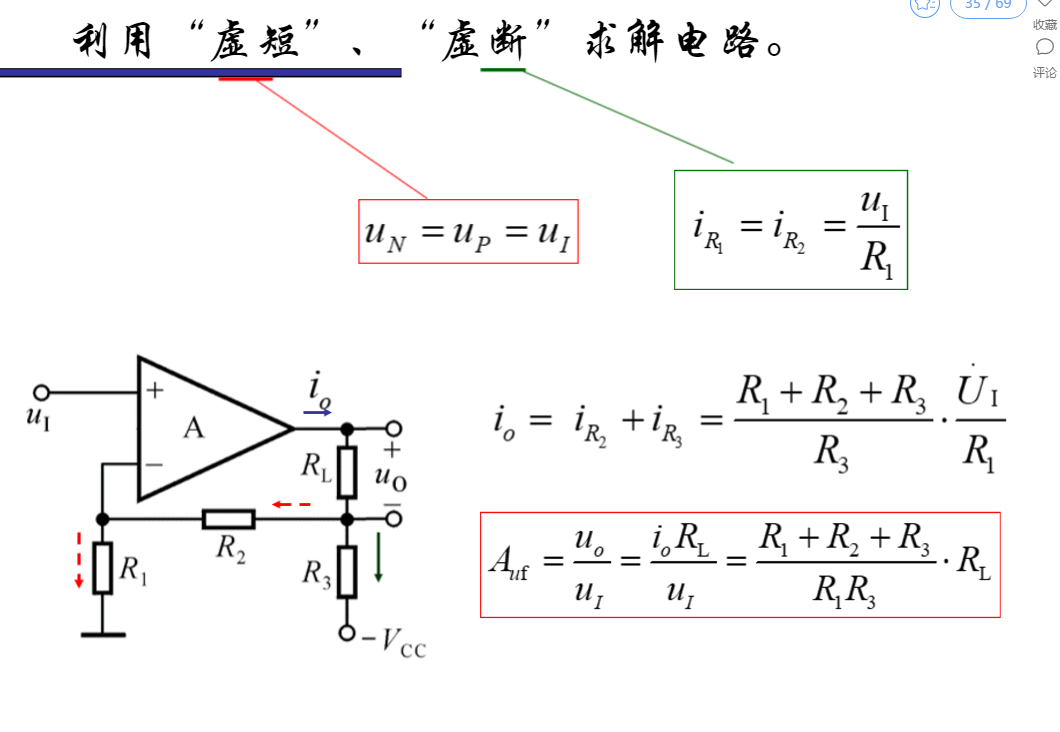展开全文• 1.利用 关系式 A˙f≈1F˙\dot{A}_f \approx \frac{1}{\dot{F}}A˙f​≈F˙1​ 估算闭环电压放大倍数。 注意 ： 只适合于电压串联负反馈组态。 A˙uuf≈1Fuu˙\dot{A}_{uuf} \approx \frac{1}{\dot{F_{uu}}}A˙uuf...

1.基础公式与结论

• 1.利用 关系式 A ˙ f ≈ 1 F ˙ \dot{A}_f \approx \frac{1}{\dot{F}} 估算闭环电压放大倍数。

• 注意 ： 只适合于电压串联负反馈组态。
• A ˙ u u f ≈ 1 F u u ˙ \dot{A}_{uuf} \approx \frac{1}{\dot{F_{uu}}}
• 1.求反馈电压 U ˙ f \dot{U}_f
• 2.求反馈系数 F ˙ u u = U ˙ f U ˙ o \dot{F}_{uu} = \frac{\dot{U}_f}{\dot{U}_o}
• 3.得出闭环电压放大倍数 A u u f ≈ 1 F ˙ u u A_{uuf} \approx \frac{1}{\dot{F}_{uu}}
• 2.利用 关系式 X ˙ f ≈ X ˙ i \dot{X}_f \approx \dot{X}_i 估算闭环电压放大倍数。

• 注意 ： 适合于除 电压串联负反馈 之外的三种组态。
• 串联负反馈 ： U ˙ f ≈ U ˙ i \dot{U}_{f} \approx \dot{U}_{i}
• 并联负反馈 ： I ˙ f ≈ I ˙ i \dot{I}_{f} \approx \dot{I}_{i}
• 1.通过上述关系得出闭环电压放大倍数。

2.教材标准案例• 1.图(a)为电压并联负反馈，且在深度负反馈电路中，利用 关系式 I ˙ f ≈ I ˙ i \dot{I}_f \approx \dot{I}_i 估算。在开环增益足够大的情况下，可认为反相输入端的电压近似为零，则求得$\dot{I}_i 和 和 \dot{I}_f$
I ˙ i ≈ U ˙ i R 1 \dot{I}_i \approx \frac{\dot{U}_i}{R_1}
I ˙ f ≈ − U ˙ o R F \dot{I}_f \approx -\frac{\dot{U}_o}{R_F} // 电流流向相反
I ˙ f ≈ I ˙ i \dot{I}_f \approx \dot{I}_i ，得
− U ˙ o R F ≈ U ˙ i R 1 -\frac{\dot{U}_o}{R_F} \approx \frac{\dot{U}_i}{R_1}
则闭环电压放大倍数为
A ˙ u f = U ˙ o U ˙ i ≈ − R F R 1 = − 2.2 20 = − 0.11 \dot{A}_{uf} = \frac{\dot{U}_o}{\dot{U}_i} \approx -\frac{R_F}{R_1} = - \frac{2.2}{20} = -0.11

• 2.图(b)为电压串联负反馈，利用 关系式 A ˙ u u f ≈ 1 F u u ˙ \dot{A}_{uuf} \approx \frac{1}{\dot{F_{uu}}} 估算。
U ˙ f = R 3 R 2 + R 3 U ˙ o \dot{U}_f = \frac{R_3}{R_2 + R_3}\dot{U}_o
∴ \therefore
F ˙ u u = U ˙ f U ˙ o = R 3 R 2 + R 3 \dot{F}_{uu} = \frac{\dot{U}_f}{\dot{U}_o} = \frac{R_3}{R_2 + R_3}

A ˙ u f ≈ 1 F ˙ u u = 1 + R 2 R 3 = 1 + 3 2 = 2.5 \dot{A}_{uf} \approx \frac{1}{\dot{F}_{uu}} = 1 + \frac{R_2}{R_3} = 1 + \frac{3}{2} = 2.5

• 3.图（c）为电流并联负反馈，利用 关系式 I ˙ f ≈ I ˙ i \dot{I}_{f} \approx \dot{I}_{i} 估算。
I ˙ i ≈ U ˙ i R ˙ 1 \dot{I}_i \approx \frac{\dot{U}_i}{\dot{R}_1}
I ˙ f ≈ − I ˙ o R 3 R 3 + R F = − U ˙ o R L ⋅ R 3 R 3 + R F \dot{I}_f \approx -\frac{\dot{I}_oR_3}{R_3 + R_F} = -\frac{\dot{U}_o}{R_L}·\frac{R_3}{R_3 + R_F}

− U ˙ o R L ⋅ R 3 R 3 + R F ≈ U ˙ i R ˙ 1 -\frac{\dot{U}_o}{R_L}·\frac{R_3}{R_3 + R_F} \approx \frac{\dot{U}_i}{\dot{R}_1}
∴ \therefore
A ˙ u f = U ˙ o U ˙ i ≈ − R L ( R 3 + R F ) R 1 R 3 = − 2 ( 5.1 + 10 ) 1 × 5.1 = − 5.92 \dot{A}_{uf} = \frac{\dot{U}_o}{\dot{U}_i} \approx -\frac{R_L(R_3 + R_F)}{R_1R_3} = -\frac{2(5.1 + 10)}{1 \times 5.1} = -5.92

• 4.图(d)为电流串联负反馈，利用 关系式 U ˙ f ≈ U ˙ i \dot{U}_{f} \approx \dot{U}_{i} 估算。
U ˙ f = I ˙ o R F ≈ U ˙ i \dot{U}_f = \dot{I}_oR_F \approx \dot{U}_i

U ˙ o = I ˙ o R L \dot{U}_o = \dot{I}_oR_L

A ˙ u f = U ˙ o U ˙ i ≈ R L R F = 5.1 15 = 0.34 \dot{A}_{uf} = \frac{\dot{U}_o}{\dot{U}_i} \approx \frac{R_L}{R_F} = \frac{5.1}{15} = 0.34

3.总结一下罢

• 前提 ：负反馈

• 电压并联

• 利用 关系式 I ˙ f ≈ I ˙ i \dot{I}_f \approx \dot{I}_i 估算
• 电压串联

• 利用 关系式 A ˙ u u f ≈ 1 F u u ˙ \dot{A}_{uuf} \approx \frac{1}{\dot{F_{uu}}} 估算
• 电流并联

• 利用 关系式 I ˙ f ≈ I ˙ i \dot{I}_{f} \approx \dot{I}_{i} 估算
• 电流串联

• 利用 关系式 U ˙ f ≈ U ˙ i \dot{U}_{f} \approx \dot{U}_{i} 估算
展开全文经验分享
• 电子编程与技术入门总目录6.1 运算放大器 开环 闭环 反馈反馈反馈 虚断 虚短知乎向导电子编程专栏总目录：最好的电子、计算机从入门到工程师教程知乎向导博客提示一下,实际上大家看本课应该从6.1.5开始看，也...

第六章 实用运放电路与电源电路

以书籍格式、难易程度重新编排文档...

电子编程与技术入门总目录

6.1 运算放大器 开环 闭环 反馈 正反馈 负反馈 虚断 虚短

知乎向导电子编程专栏总目录：最好的电子、计算机从入门到工程师教程

知乎向导博客

提示一下,实际上大家看本课应该从6.1.5开始看，也就是倒推法！

部分图后补。

6.1.1 运算放大器

昨天一位同学连续给我提了几个问题，就是这节的题目，越来越感觉到课程不能跳跃式的讲，初学者所有的问题都是问题。一旦牵扯到一个专业的名词，都有必要把它的核心含义、适用条件说说，这样才能不迷糊的往下进行。

需要知道这个名词，也要知道这个名词的用处和使用范围，并且将应用电路涉及的基本名词一起解释才容易理解！

如果是学电的朋友，先学的是三极管的放大电路，后面学的运算放大器电路，实际上三极管放大电路讲课时都很少去讲它，原因？ 是因为三极管放大电路不稳定，实际上现在已经大部分情况都被运算放大器电路替代了。

运算放大器（后续简称运放）是一种集成电路，内部有很多三极管类晶体管的组合。外围接很少的电子元器件就能够实现放大信号的作用，并且信号干净、漂亮、准确... 好处一箩筐，就犹如年轻人用了智能手机，你还想回去用只能打电话的手机吗？

现在大部分电路都是数字电路，模拟电路一般都被模块化，可以说理解了运放和电源电路，那么你就是一个水平不低的电子工程师。

模拟电路和数字电路后讲，这里带出这两个名词，是说明运放的重要性。

运放电路是模拟电路的王者之一，甚至可以去掉之一这个限定词!

后面的这些开环、闭环、反馈、正反馈、负反馈、虚断、虚短的名词本课是为了分析两种基本的运放电路输入输出电压公式服务。

6.1.2 开环 闭环

我们只针对运算放大器电路来讲开环、闭环。

开环电路是不接入反馈的电路，输出端不会影响到输入端。

闭环电路是接入反馈的电路，也就是输出端会影响到输入端。

那么对运放来说，接入反馈电阻（或其他电子器件）是闭环电路，不接入是开环电路。

基本的运放电路都是闭环电路，这是最通常的应用！

6.1.3 反馈 正反馈 负反馈

反馈实际上就是把后级的电流电压信号，通过一定路径折返回前级电路。

如果这个返回的信号，使输入信号（前级电路信号）变大，那么就是正反馈，如果使输入信号变小，就是负反馈

这里还是针对运放来说，

基本的运放电路大部分情况都是要求接入负反馈，这是最通常的应用！

6.1.4 虚短 虚断

先说为什么产生这两个名词，我们再去解释它！

运放内部电路非常复杂，分析起来相当麻烦，为了大部分应用工程师，而不是做科研的工程师服务，也为了初学者容易使用运放发明了这两个名词。

为了使输入电压、输出电压的公式计算易于理解，并且在绝大部分情况下这样计算就行，就利用虚短、虚断来分析计算电路。

这是运放两个基本特性。

虚断：运放的两个输入端，内部阻抗（电阻）非常大，大到只有很微弱的电流能够流进运放内部。看起来几乎就是完全断掉的电路一样。 我们分析运放电路时，这两个输入端就可以理解为直接断开就行。这是运放本身的设计决定的，自带属性。两个输入端可以认为无电流输入。

虚短：短的意思是相当于一根电线把运放两个输入端直接连一块，也就是分析时，两个输入端的电压可以认为相等。

虚短有两个限定条件：

1. 运放搭成开环电路，运放工作在线性区间
2. 运放搭成闭环电路，运放工作在深度负反馈状态

这里解释下，上面第一个很少使用，假设使用也是很固定的几种模式，完全可以不用深入理解。 第二个可以通过实例去理解，运放能够正常工作起来必然是深度负反馈，所以你可以直接把深度去掉。

6.1.5 反向运放、同向运放的虚短、虚断的分析应用。

名词的出现是为了用的！

反向运算放大器电路：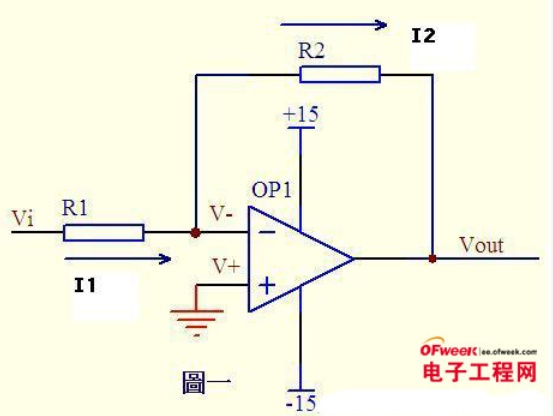建立条件：

• 接上了R2 作为反馈电阻，运行起来后，此运放进入深度负反馈，所以运放符合虚短的情况。
• 本身运放就是虚断的，所以虚短、虚断都成立。
• Vi这个电压信号从运放的负端输入，所以这个电路是反向运算放大器，输出与输入信号方向相反。

利用虚短、虚断推导反向运放输出电压计算公式：

1. 虚短，两个输入端电压相等， 所以 V+ = V- = GND（看地符号）=0V
2. 虚断，V- 的电流是流不进运放的，因为阻抗大到电流忽略不计的程度，所以V-到运放相当于断开。所以电流从 Vi 到 v- 再到 R2 再到 Vout，而不经过运放（注意这样分析，实际必然有一点电流流进运放的，不然运放怎么工作，但是计算时这个电流可以忽略掉）
3. 根据1.4节的基尔霍夫电流定律我们可以知道，流入节点的等于流出的电流。因为V-到运放内部的电路是虚断的，所以没电流，这样电流I1 到了 V- 位置，和从 V- 流出的电流 I2 是相等的，所以我们得出 I1=I2
4. I1=I2, 然后根据最基本的欧姆定律（1.4）电流=电压/电阻，我们得出 (Vi - (v-))/R1 = ((v-)-Vout)/R2
5. 我们根据第一步 虚短概念知道，V- = 0V，所以得到 (Vi-0)/R1 = (0-Vout)/R2
6. 最后我们得到，Vi/R1 = -Vout/R2, Vout=-Vi*R2/R1,这就是最基本的反向运放放大倍数计算公式。

反向运放计算公式：Vout=-Vi*R2/R1

===================

同向运算放大器电路：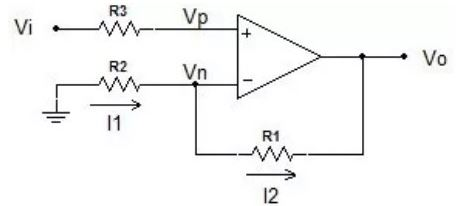建立条件：

• 接上了R1 作为反馈电阻，运行起来后，此运放进入深度负反馈，所以运放符合虚短的情况。
• 本身运放就是虚断的，所以虚短、虚断都成立。
• Vi这个电压信号从运放的正端输入，所以这个电路是同向运算放大器，输出与输入信号方向相同。

利用虚短、虚断推导同向运放输出电压计算公式：

1. 虚断，Vp 的电流是流不进运放的，我们知道断路的电路，断掉的地方电压就是输入的电压，所以Vp = Vi。
2. 根据1.4节的基尔霍夫电流定律我们可以知道，流入节点的等于流出的电流。因为 Vn 到运放内部的电路是虚断的，所以没电流，这样电流I1 到了 Vn 位置，和从 Vn 流出的电流 I2 是相等的，所以我们得出 I1=I2
3. I1=I2, 然后根据最基本的欧姆定律（1.4节）电流=电压/电阻，我们得出 (0-Vn)/R2 = (Vn-Vo)/R1
4. 我们根据虚短概念知道，Vp=Vn，再根据第一步虚断推论，得到Vp=Vi=Vn，所以得到 （0-Vi)/R2 = (Vi-Vo)/R1
5. 最后我们得到，Vo=(1+(R1/R2))*Vi, 这就是最基本的同向运放放大倍数计算公式。

同向运放计算公式： Vo=(1+(R1/R2))*Vi

总结下：

我们了解很多名词是为了什么？ 是为了简化计算，为后面的推导建立条件。 实际作为应用工程师，你完全可以避开这些概念，直接使用最后推导出的公式。 我们在什么时候用到这个推导，分析其他人的电路和应聘、考试上。

学和用是两个阶段，学以致用，有些东西上学阶段是重要的，应聘时也是重要的，但是我们去用可能很简单，所以不要被眼花缭乱的公式推导吓住，真的做到了电子工程师，你并不一定需要到去推导，因为是团队作战，也是站在前辈的基础上工作，很多复杂的步骤已经被标准化了，拿来就用是可以的。

并不是所有概念都搞懂才能做研发、才可以发明创造，而是了解大部分基本原理就可以，你为什么不能去做呢？

本来就是拿着应用工程师的薪资，非要用科研工程师的紧箍咒往头上套，这就是自找麻烦了。

脑力够就继续深入学习，脑力欠佳就学会应用，一样可以对得住自己的努力！

一定的工作范围内，只会应用的工程师也是合格的工程师！

参考：

反向运放电路通过虚短、虚断进行电路分析：

运放的虚短、虚断

同向运放电路通过虚短、虚断进行电路分析：

记住这几个特性，运算放大器的使用不再难

如果觉得有用给点个赞，或者评论下，让更多的人受益。感谢！

声明：

文中所有论点、结论皆根据作者经历经验总结，限于知识结构、层次、经历局限性，必然有不少偏颇，甚至部分内容偏激，作者所有的文章也是试错的过程，欢迎批评斧正，如有不同意见或建议，请与讨论区提出讨论，致谢！

展开全文• 本节内容主要从负反馈放大电路的方框图、放大倍数的一般表达式、深度负反馈等来进行介绍！ 负反馈放大电路方框图 说明负反馈放大电路的方框图不是简单断开反馈得到的，而是根据网络理论断开反馈经单向化后得到的；...
• 运放参数中的增益多指运放的最大放大倍数(视反馈电阻无穷大)。dB是分贝，它与倍数有一个对数的换算关系。假设放大倍数为A倍，那么可以说放大倍数为20lgA分贝。例如放大倍数为1000倍，根据公式换算后可以说放大倍数为...电压放大倍数公式运放
• 本文旨在学习如何快速简单地对运算放大器进行分析;1 运算放大器(OPAMP)2 虚短和虚断3.1 典型电路3.2 放大倍数3.3 仿真结果4.1 双...，集成运放最终放大的是差模信号，在没有引入反馈的情况下，电压的放大倍数为差...
• 运算放大器在信号的采集、放大等各种应用中非常广泛，其应用电路也非常多，因此我们特地针对运算...理想的运算放大器其开环差模放大倍数为∞。也就是说，即使两输入端加上无穷小的输入电压，也足以让运放工作在非线...
• 增益K计算公式如下：K = 1 + Rf / Rs同相放大器，施加的反馈方式是电压串联负反馈，这种负反馈具有增大输入电阻、降低输出电阻的作用。然而，反馈深度又决定了输入电阻、输出电阻的改变程度。当...
• 第17讲 负反馈放大电路方框图及放大倍数估算.ppt
• 虽然它使放大器的放大倍数降低，但能在多方面改善放大器的动态指标，如稳定放大器的放大倍数，改变放大器的输入电阻和输出电阻，减少非线性失真和展宽通频带等。因此，几乎所有的实用放大器都引入负反馈
• ▌1.题目设计一个有三极管构成的电流负反馈放大器，放大倍数不小于100倍，集电极最大电流不超过20mA，当负载由1000欧姆变10欧姆时，要求输出电流的波动幅度小于5%。▌2.项目总体功能...adb 传感器 嵌入式 html verilog
• 开环放大倍数与闭环放大倍数，这两个的区别在于：控制的精度不同。举个简单的例子吧，让您去送快递，只是给您下达了这样一个指令，但快递是否送到了，这个东东是无从得知的，这就是开环；假如让您去送快递，而且要让...
• 1.一般反相/同相放大电路中都会有一个平衡电阻，这个平衡电阻的作用是什么呢?(1) 为芯片内部的晶体管提供一个合适的静态偏置。芯片内部的电路通常都是直接耦合的，它能够自动调节静态工作点，但是，如果某个输入引脚...
• 知道2点电压,下面再球6点电压,即输出电压.U6/(R3+R4)=U2/R3,这样就得到放大倍数：U6/Ui=(R3+R4)*R1/(R1+R2)*R3./*************/U2<U6,6端R3所在的接地端组成的回路分析运放负反馈电路,最关键要常握好虚短和虚断这...
• 放大器分类及放大倍数的计算放大电路种类电压跟随器电压放大器负反馈电压放大器正反馈电压放大器加法器同相加法器反相加法器差分放大电路放大电路的接法共射极放大电路共基极放大电路共集电极放大电路 首先我们来讲...放大器 加法器
• 反馈放大器 在放大器中采用负反馈电路，其目的是为了改善放大器的工作性能，提高放大器的输出信号质量。在引入负反馈电路之后，放大器的增益 要比没有负反馈时的增益小，但是可以改善放大器的许多性能，主要有四...
• 运算放大器的基础原理运算放大器具有两个输入端和一个输出端，如图1-1所示，其中标有“+”号的输入端为“同相输入端”而不能叫做正端)，另一只标有“一”号的输入端为“反相输入端”同样也不能叫做负端，如果先后...
• 噪声是电子设计中必须处理等信号之一，我们都知道放大器的噪声有两种类型：一种是外部噪声，来源于放大器外部；另一种是内部噪声，来源于器件本身，处理放大器的噪声对于提升电子产品的性能至关重要，这里我们以问答...
• 1.已知放大电路的放大倍数，若放大信号为电压信号，要看传感器或输入设备的内阻。要达到高输入阻抗的效果。选择时要在运放的输入端加上远大于设备内阻的阻值电阻。 2.已知放大电路的放大倍数，若放大信号为电流信号...
• 测定两级电压串联负反馈电路　在开环（8连9可近似地认为得出了没有负反馈作用但有反馈网络负载效应的基本放大器S）与闭环时（7连9）中频段电压放大倍数。 表一 静态工作点的测量 　从输入端输入1 kHz约5 mV的正弦...
• 运放电路放大倍数的计算

千次阅读 2020-12-22 18:17:22
展开全部在求分立元件多级放大32313133353236313431303231363533e4b893e5b19e31333431363535电路的电压放大倍数时有两种处理方法。一是将后一级的输入电阻作为前一级的负载考虑，即将第二级的输入电阻与第一级集电极...电压放大倍数公式运放
• 运算放大器，运放，在电路设计中非常常见。通过运放，能够实现很多复杂的电路，但也因此成为最难理解的电子元器件。在此，我们以LM741作为例子，学习运放的基本特性。一、运放ICLM741应该是史上最为成功的IC之一了。...
• 原文链接：运算放大器基本电路大全原文作者：电子工程专辑引言我们经常看到很多非常经典的运算放大器应用图集，但是这些应用都建立在双电源的基础上，很多时候，电路的设计者必须用单电源供电，但是他们不知道该如何...
• 模拟电子技术基础：第17讲 负反馈放大电路方框图及放大倍数估算.ppt
• 通常引入反馈深度越大，对于电路性能的改善越好，如增益稳定性的提高，通频带的展宽，非线性失真的减小，输入电阻的增加和输出电阻的减小。但是，反馈深度越大，对电路的增益衰减也越大，所以负反馈是以牺牲增益为...
• 深度负反馈放大电路放大倍数的分析 深度负反馈的实质 负反馈放大电路的一般表达式中：
• 虚短虚断是解开负反馈运放电路的核心方法，具体就要从运放的原理开始说起了。LM741原理图拿LM741为例子。可以将内部电路图分为A,B,C,D四个部分。A是差分输入级。差分输入有同相输入和反相输入两个输入端。主要作用是...
• 虚短和虚断的概念 由于运放的电压放大倍数很大，一般通用型运算放大器的开环电压放大倍数都在80 dB以上。而运放的输出电压是有限的，一般在 10 V～14 V。因此运放的差模输入电压不足1 mV，两输入端近似等电位，相当......